# Electronics and Communication Engineering - Electromagnetic Field Theory

41.
Calculate cut off frequency for dominant mode in rectangular waveguide if dimensions are a = 2, b = 1 cm.
7.5 GHz
1.5 GHz
15 GHz
150 GHz
Explanation: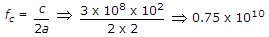7.5 x 109 Hz7.5 GHz.

42.
The input impedance for a half dipole if radii of both the antenna is 4 and 8 meter respectively
600 Ω
100 Ω
657 Ω
650 Ω
Explanation: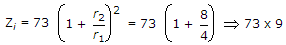657 Ω.

43.
A rectangular waveguide measures 3 x 4.5 cm internally and has a 10 GHz signal propagated in it. Calculate the guide wavelength for dominant mode.
13.5 m
3 m
88/3 m
3/88 m
Explanation: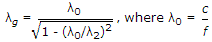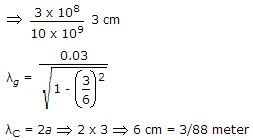.

44.
A rectangular waveguide measures 3 x 4.5 cm internally and has a 10 GHz signal propagated in it. Calculate the cut off frequency for dominant mode.
4 GHz
7.5 GHz
5 GHz
10 GHz
Explanation: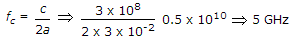.

45.
Find the flux crossing the plane surface defined by 0.5 ≤ r ≤ 3.5 m and 0 ≤ z ≤ 3 m. if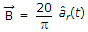0
255 Wb
6.45 Wb
none of the above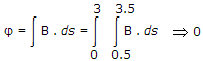.